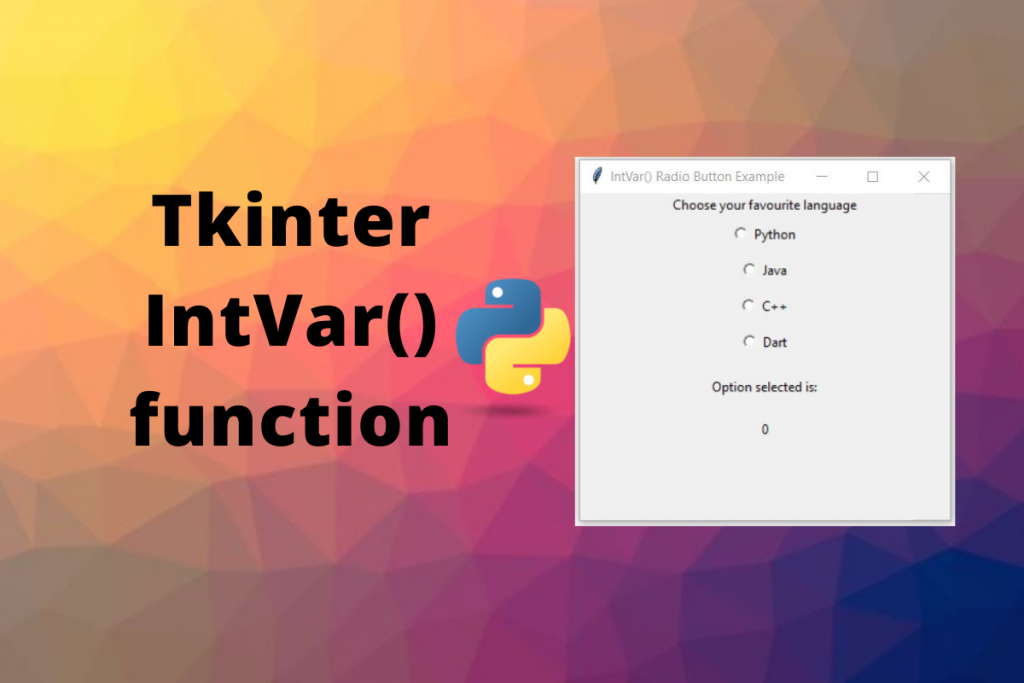# Tkinter IntVar – Tkinter Tutorial with ExamplesHello everyone! In this tutorial, we will learn about the Tkinter IntVar function and how to use it to store, retrieve and manage integer variables in Python. So let’s get started with IntVar() function.

## Tkinter IntVar() Function

Tkinter contains built-in programming types which work like a normal python type with additional features used to manipulate values of widgets like `Label` and `Entry` more effectively, which makes them different from python data types. These variables also contain getter and setter methods to access and change their values. `IntVar` is an example of them.

A variable defined using `IntVar()` function holds integer data where we can set integer data and can retrieve it as well using getter and setter methods. These variables can be passed to various widget parameters, for example, the variable parameter of Radio Button and CheckBox Button, textvariable parameter of Label Widget, etc as we will see in examples. Once these variables get connected to widgets, the connection works both ways: if the IntVar() variable changes, the value of the widget also gets updated automatically with the new value.

## Defining The Tkinter IntVar() Variable

We need Tkinter `IntVar()` function which takes the following parameters to define the variable:

• master: It is the window with which `IntVar()` variable is associated. If nothing is specified, it defaults to root window.
• value: The initial value given to the integervariable. Defaults to 0.
• name: The name given to the defined variable. Default value is PY_VARnum(like PY_VAR1, PY_VAR2, etc).
```import tkinter as tk

master_window = tk.Tk()
master_window.geometry("250x150")
master_window.title("IntVar Example")

integer_variable = tk.IntVar(master_window, 255)

label = tk.Label(master_window, textvariable=integer_variable, height=250)
label.pack()

master_window.mainloop()
```

## Changing Values Of IntVar() Variables

Since `IntVar()` is Tkinter built programming type, it contains setter method to change values of their variables. We can use `set()` method to change the values of integer data.

```import tkinter as tk

master_window = tk.Tk()
master_window.geometry("250x150")
master_window.title("IntVar Example")

integer_variable = tk.IntVar(master=master_window, value=1)

label = tk.Label(master_window, textvariable=integer_variable, height=250)
label.pack()

integer_variable.set(100)

master_window.mainloop()
```

## Retrieving Values Of IntVar() Variables

We can use `get()` method on `IntVar()` variable to retrieve the text value present in the variable.

```import tkinter as tk

master_window = tk.Tk()

int_var = tk.IntVar(master = master_window, value = 255)

num = int_var.get()

print(type(num))
print(num)
```

Output:

```<class 'int'>
255
```

## Tkinter IntVar() Examples

Let’s consider some examples of different usages of IntVar().

### Notify When IntVar() Variable Data Changes

We can get notified and do some tasks whenever the value of `IntVar()` variable changes. This is one of the interesting features of Tkinter defined object to be notified whenever its value of read, updated or deleted. This feature is also helpful if you want to update other widgets automatically in case of some operations on `IntVar()` variables.

To define a callback on `IntVar()` object, we can use `trace()` method on the `IntVar()` object that takes 2 parameters:

• mode: The type of operation on the `IntVar()` object.
• `'write'`: invoke callback when value is changed
• `'read'`: invoke callback when value is read
• `'unset'`: invoke callback when value is deleted
• callback: method to call when there is an operation on the object.

Let’s consider a simple example of the sum of 2 numbers, where we have 2 Entry widgets to take input of numbers and a label that displays the sum of 2 numbers. The sum will be updated automatically whenever the Entry widget and hence the `IntVar()` variable changes.

```import tkinter as tk

class SumOfTwoNumbers(tk.Tk):
def __init__(self):
super().__init__()
self.title("Sum of 2 Numbers")
self.geometry("300x300")

# define IntVar() variables A and B
self.A = tk.IntVar()
self.B = tk.IntVar()

# assign methods to notify on IntVar() variables

self.create_widgets()

def create_widgets(self):
self.A_label = tk.Label(self, text="A: ")
self.B_label = tk.Label(self, text="B: ")

self.A_entry = tk.Entry(self, textvariable=self.A)
self.B_entry = tk.Entry(self, textvariable=self.B)

self.sum_label = tk.Label(self, text="Sum: ")
self.result_label = tk.Label(self, text=self.A.get() + self.B.get())

def calculate_sum(self, *args):
try:
num_a = self.A.get()
except:
num_a = 0

try:
num_b = self.B.get()
except:
num_b = 0

self.result_label['text'] = num_a + num_b

if __name__ == "__main__":
app = SumOfTwoNumbers()
app.mainloop()
```

Its output:

### Monitoring Selected Value In RadioButton And CheckBox Widget

Another common application of `IntVar()` variable is to track the value selected in RadioButton and CheckBox Widget as shown in the following example:

```import tkinter as tk

def __init__(self):
super().__init__()
self.geometry("300x300")

# define IntVar() for selected value
self.selected_value = tk.IntVar()

self.create_widgets()

def create_widgets(self):
self.intro_label = tk.Label(
self,

self,
text="Python",
variable=self.selected_value,
value=1).pack()

self,
text="Java",
variable=self.selected_value,
value=2).pack()

self,
text="C++",
variable=self.selected_value,
value=3).pack()

self,
text="Dart",
variable=self.selected_value,
value=4).pack()

self.text_label = tk.Label(
self,
text="Option selected is:",
).pack()

self.value_label = tk.Label(
self,
textvariable=self.selected_value,
In this tutorial, we learned about Tkinter `IntVar()` and various scenarios where we can use it and it can make our lives easier but defining callbacks that can help us to automatically update other widgets.